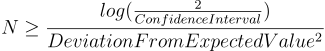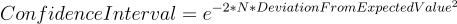## Hoeffding's Inequality

Hoeffding's Inequality is a beautiful inequality used to calculate how far a true, underlying, statistic of a population could deviate from some observed value collected from an IID sample under a given confidence interval.

The confidence interval

The expected value (E[x])

Variable range width

The x axis lower and upper limitsThe number of samples you have

The expected value (E[x])

Variable range width

The x axis lower and upper limits